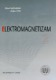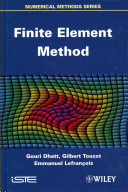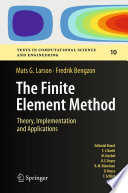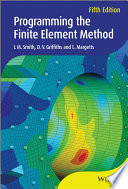### Finite Element Method Programming

#### Course Description

Finite element method (FEM) provide a general and powerful framework for solving ordinary and partial differential equations. It is particularly useful for engineering problems that are too complicated to be solved by classical analytical methods. In this course, we introduce approximation with finite elements, discretisation via weak form formulation of various engineering differential equations, piecewise polynomial approximation, quadrature, and triangulation in one, two and three space dimensions. Students will be introduced to programming techniques for computer implementation of the method in Matlab, Mathematica and Python environments. Introduction, nodal approximation, approximation with finite elements, geometrical definition of elements, approximation based on a reference element Construction of shape functions, transformation of differential operators, computation of shape functions, their derivatives and Jacobian matrix, truncation errors in an element Various Types of elements: line elements, triangular elements, quadrilateral elements Three-dimensional elements: tetrahedrons, hexahedrons, prisms, other elements Variational formulation of Engineering problems, weighted residual method, functionals Discretization of integral forms, choice of weighting functions, properties of the system of equations Matrix formulation of FEM, discretized integral forms, computational techniques for element matrices Numerical procedures, integration in one, two and three dimensions, precision of integration, numerical codes Solution of system of linear equations, Gaussian elimination, decomposition, sparse systems Non-linear systems, substitution method, Newton-Raphson method, incremental method, solution strategy, convergence Programming techniques, functional bocks of a finite element program, description of a typical problem General programs, description of the finite element code, library of the finite element method Examples of application, heat transfer, static electric fields, fluid flow

#### Learning Outcomes

1. Explain approximation with finite elements
2. Apply various types of elements in the approximation.
3. Explain variational formulation of engineering problems
4. Explain matrix formulation of the finite element method
5. Apply various numerical procedures in the finite element method
6. Apply programming techniques for the finite element method

#### Forms of Teaching

Lectures

participation in lectures

Seminars and workshops

project and team work

Independent assignments

project and team work

Laboratory

project and team work

Work with mentor

support in team work

Continuous Assessment Exam
Seminar/Project 0 % 30 % 0 % 30 %
2. Mid Term Exam: Written 0 % 30 % 0 %
Final Exam: Written 0 % 30 %
Final Exam: Oral 10 %
Exam: Written 0 % 60 %
Exam: Oral 10 %

#### Week by Week Schedule

1. Variational methods
2. Variational Formulation of the Elliptic Linear Model Problem
3. The Finite Elements in One-dimensional Space; Linear Finite Elements; Interpolation Operator and Interpolation Error
4. The Finite Elements in Two-dimensional Space; Triangulation of the Domain; The Finite Element Space; Lagrange Finite Elements
5. Discretization of weak formulation; Numerical integration
6. Formation of local matrix in a finite element; Assembling global matrices over a problem domain
7. Analysis of the obtained matrix equations: symmetry and density of matrices; Direct and iterative methods for solving matrix equations: numerical stability and convergence
8. Midterm exam
9. The Finite Elements in Three-dimensional Space
10. Convergence of Conforming Methods
11. Finite Element Method for Unsteady Problems: On the Weak formulation; Semi-Discretization by Finite Elements; Temporal Discretization; Error Control
12. Finite Element Method for Unsteady Problems: On the Weak formulation; Semi-Discretization by Finite Elements; Temporal Discretization; Error Control
13. Nonlinear Problems and Systems
14. Nonlinear Problems and Systems
15. Final exam

#### Study Programmes

Audio Technologies and Electroacoustics (profile)
Elective Courses of the Profile (2. semester)
Communication and Space Technologies (profile)
Free Elective Courses (2. semester)
Computational Modelling in Engineering (profile)
Elective Courses of the Profile (2. semester)
Computer Engineering (profile)
Free Elective Courses (2. semester)
Computer Science (profile)
Free Elective Courses (2. semester)
Control Systems and Robotics (profile)
Free Elective Courses (2. semester)
Data Science (profile)
Free Elective Courses (2. semester)
Electrical Power Engineering (profile)
Free Elective Courses (2. semester)
Electric Machines, Drives and Automation (profile)
Free Elective Courses (2. semester)
Electronic and Computer Engineering (profile)
Free Elective Courses (2. semester)
Electronics (profile)
Free Elective Courses (2. semester)
Information and Communication Engineering (profile)
Free Elective Courses (2. semester)
Network Science (profile)
Free Elective Courses (2. semester)
Software Engineering and Information Systems (profile)
Free Elective Courses (2. semester)

#### LiteratureZijad Haznadar, Željko Štih (1997.), Elektromagnetizam 2, Školska knjigaGouri Dhatt, Gilbert Touzot, Emmanuel Lefrançois (2012.), Finite Element Method, , Inc,UK, 2012, Wiley-ISTEMats G. Larson, Fredrik Bengzon (2013.), The Finite Element Method: Theory, Implementation, and Applications, SpingerIan M. Smith, D. V. Griffiths (2004.), Programming the finite element method, Wiley

#### General

ID 222724
Summer semester
5 ECTS
L3 English Level
L2 e-Learning
30 Lectures
13 Laboratory exercises